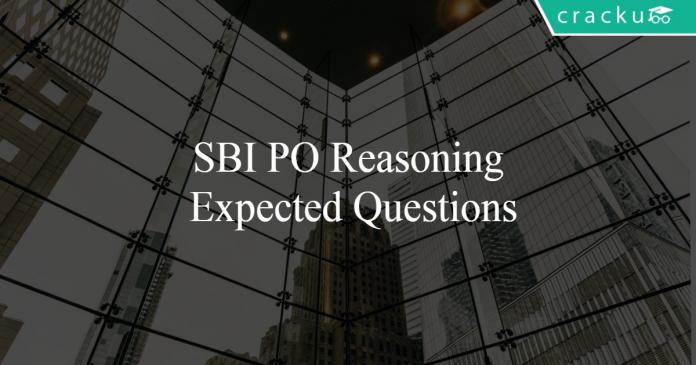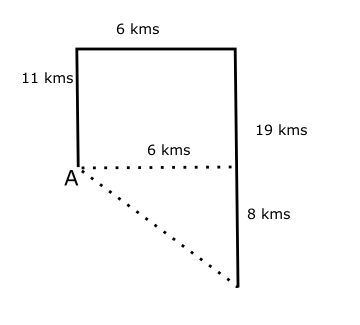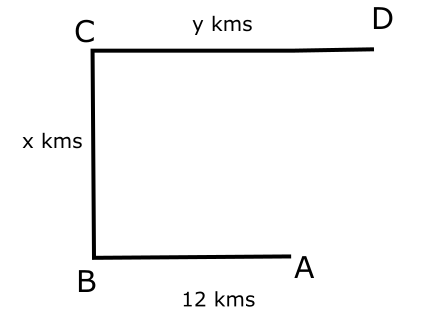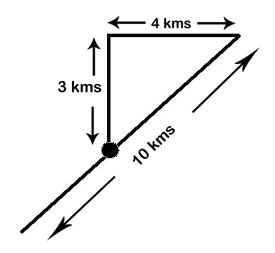# SBI PO Reasoning Expected Questions

0
160Download SBI PO Expected Reasoning Questions & Answers PDF for SBI PO Prelims and Mains exam. Very Important SBI PO Expected Reasoning questions with solutions.

Question 1: An embryo is related to a new-born in the same way as ………………..

a) an egg is related to a chick

b) a student is related to a teacher

c) an old man is related to a young man

d) a lion is related to a lioness

Question 2: 1992 : 996 :: ? : 448

a) 894

b) 786

c) 224

d) 896

Question 3: In a certain encryption “CARDIAC” is coded as “ACRDICA”. How is “READER” coded in the same encryotion?

a) REARED

b) REDAER

c) ERDEAR

Question 4: In a certain encryption, “PETALS” is coded as “UJYFQX”. How is “MASTER” coded in the same encryption?

a) GYKHTG

b) PJGEGH

c) USJAKIL

d) RFXYJW

Question 5: 7 : 51 :: 5 : ?

a) 23

b) 24

c) 25

d) 27

Question 6: For which of the following options, the conclusion $F \leq D$ will be definitely false?

a) $F= E \leq B \leq A \leq D$

b) $F= E \leq B \geq A \leq D$

c) $F= E \geq B > A \geq D$

d) $F= E > B \leq A\leq D$

e) More than one of the above

Question 7: For which of the following options, the conclusions $A\geq B$ and $C < D$ will definitely hold true?

a) $D > A \geq E = C \geq B$

b) $D > A \geq E < C < B$

c) $D \geq A = E < C > B$

d) $D \geq A > E > C = B$

e) $D \leq A > E < C = B$

Question 8: Select the option that will fill the blanks such that the conclusion $P \leq Q$ is definitely false:
P_____A_____R_____F_____Q

a) $=, \leq , \leq, =$

b) $= , \geq, = , >$

c) $\geq, \leq, = ,>$

d) $>, > , < , =$

e) $\geq, =, <, \geq$

Question 9: Select the option that will fill the blanks such that the conclusion $D<A$ is definitely true.
A ____ C _____ B____ E_____D

a) $= , \geq, = , \leq$

b) $=, \geq, >, =$

c) $\geq, \geq, \leq, >$

d) $\geq , >, \geq, =$

e) More than one of the above

Question 10: In which of the following expressions either F > Z or F = Z is definitely true?

a) D = Z < M = T $\geq$ D = F

b) Q > F < N = K $\geq$ Z < R

c) F $\geq$ H = U $\leq$ Z < M = P

d) E > N = E < F $\leq$ R > Z

e) E < F $\geq$ J = Y = C $\geq$ Z

Question 11: Ram starts from point A and travels towards north for 11 kms and he takes right turn to travel for 6 kms and then he again takes right turn to travel for 19 kms. How far is he from the starting point A?

a) 12

b) 5

c) 8

d) 10

e) none of these

Question 12: Ram starts from point A and rides towards the west for 12 kms to reach B, then he takes right turn and travel for ‘x’ kms to reach point C and then he takes another right turn and travel for y kms to reach point D. What is the direction he is finally facing?

a) North

b) South

c) East

d) West

e) Cannot be determined

Question 13: Ram is standing to the West of Shyam. Shyam is standing to the North of Vaibhav. Vaibhav is standing to the SouthEast of Kuru. In which direction does Kuru have to look to find Ram?

a) North East

b) North West

c) South East

d) South West

e) Can’t be determined

Question 14: Shweta started walking ten kilometers from her original point and then took a right turn. She then walked five kilometres. She then turns right and walks five kilometres. She has to look towards South West to find the original point. In which direction was Shweta initally facing?

a) North

b) North West

c) South East

d) South

e) Can’t be determined

Question 15: Ramu walks 3 kms towards North. Then he walks 4 kilometres to the East. Then he walks ten kms in the direction towards the original point. How far is he from the original point finally?

a) 3 kms

b) 4 kms

c) 5 kms

d) 7 kms

e) 10 kms

An embryo is represents a fertilized fetus before birth. An egg is similar to a fetus and a chick represents a new born.

996 = 1992/2
So, the required number = 448*2 = 896

The first two and the last two letters are interchanged keeping other letters the same.

Every letter in PETALS is replaced by a letter that is 5 places ahead in the English alphabetical series.

51 = $7^2$ + 2
So, the required number is $5^2$ + 2 = 27

First, let us eliminate the options in which there is a change in the direction of the inequality between F and D. We can eliminate option B and option D using this case.
Now, let us check options A and C.

Option A:

$F= E \leq B \leq A \leq D$
From this option, we can conclude that $F\leq D$. Therefore, the statement $F \leq D$ is definitely true.  We can eliminate option A.

Option C:

$F= E \geq B > A \geq D$
We can conclude that $F> D$. Therefore, the conclusion $F \leq D$ is definitely false in this case. Therefore, option C is the right answer.

Any option in which there is a change of sign between A and B or C and D will render the terms unrelatable. Therefore, let us first eliminate such choices.
Except options A and D, all other options involve a change in the inequality. Therefore, we can eliminate options B, C and E.
Option A:

$D > A \geq E = C \geq B$
Both the conclusions $A \geq B$ and $C<D$ hold true in this case.

Option D:
$D \geq A > E > C = B$
The conclusion $C<D$ is true but the conclusion $A \geq B$ is false in this option. Therefore, we can eliminate option D.

Therefore, option A is the right answer.

P and Q are at the either ends of the inequality. If there is an inconsistency in the sign (like change from > to < in between), no relationship can be established between P and Q. Therefore, we can eliminate options C, D and E.

Let us check options A and B.
Option A:
$P = A \leq R \leq F =Q$
We can say that $P \leq Q$ from this relation. Therefore, option A can be eliminated.

Option B:
$P = A \geq R = F > Q$
We can say with conviction that $P > Q$.Therefore, the conclusion $P\leqQ$ will be definitely false in this case. Therefore, option B is the right answer.

We can easily eliminate the options in which there is a change in the direction of the inequality between A and D, since no relationship can be established in such cases. This eliminates options A and C. Let us check options B and D.

Option B:
$A= C \geq B> E = D$
In this option, the conclusion $D<A$ is definitely true.

Option D:
$A\geq C > B\geq E = D$
In option D too, the conclusion $D<A$ is definitely true.

The conclusion $D<A$ is true for both options B and D. Therefore, option E is the right answer.

In E < F $\geq$ J = Y = C $\geq$ Z we can say that,
F $\geq$ C and C $\geq$ Z
Thus, F $\geq$ Z
Thus, option E does not definitely follow.Therefore the distance between point A and the final point is $\sqrt{8^{2} + 6^{2}}$ = 10 kms.So, the final direction faced by ram is “East”.

Just with the given information and without the exact distances between them, we can’t identify the directions between the persons because depending on the distances, the actual directions vary.Ramu walks 3 kms North and 4 kms East. So, he is at a distance of $\sqrt{3^2 + 4^2}$= 5 kms